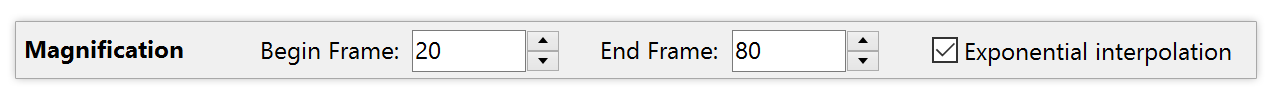# Exponential interpolation

For floating-point parameters, Ultra Fractal offers an additional interpolation setting called exponential interpolation. This can be set independently of the normal interpolation methods in the Timeline tool window.

Exponential interpolation should be used for parameters that are exponential in nature. This means that in order to experience the same apparent increase of the parameter, you need to double it each time, instead of adding something.

A perfect example is the Magnification parameter on the Location tab. It starts at 1. If you add 1 to make it 2, the fractal is magnified by a factor of two. If you add 1 again, the fractal is magnified by only a factor of 1.5. If you keep adding 1, the apparent difference gets less and less. At a magnification of 1000, you will probably not notice it if you change the magnification to 1001. In contrast, if you keep multiplying the magnification with two, you will each time experience the same zoom effect. Clearly, the Magnification parameter is exponential in nature. In contrast, the Rotation Angle parameter is linear in nature.

This is important when interpolating animations, because exponential parameters must also be interpolated exponentially. For example, if you create an animation of 99 frames where Magnification ranges from 1 at frame 1 to 16 at frame 99, it must be 4 at frame 50 to give the effect of a gradually increasing zooming level. With normal interpolation, it will be 8.5. Fortunately, the Magnification parameter uses exponential interpolation by default.To turn exponential interpolation on and off for a parameter, open the Timeline tool window, select the parameter in the tree view, and click the Exponential interpolation check box.

Notes

• Certain built-in parameters in Ultra Fractal, such as Magnification, Stretch, and Color Density in the Inside and Outside tabs, are exponential by default. When you are writing a formula, you can also specify whether or not floating-point parameters in your formula should be treated as exponential by default. See the exponential setting.
• Due to the nature of exponential values, exponential interpolation only works if both the begin and end points are nonzero and both positive or negative.